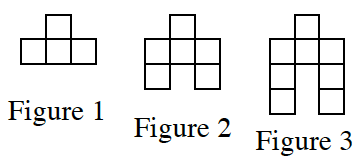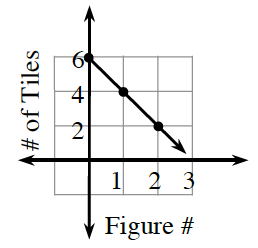### Home > CC3 > Chapter Ch6 > Lesson 6.2.6 > Problem6-109

6-109.This problem is a checkpoint for multiple representations of linear equations. It will be referred to as Checkpoint 6.

For each situation given below, complete the Representations of Patterns Web by finding the missing $x→y$ table, graph, and/or rule. Since there are many possible patterns, it is not necessary to create one.  Homework Help ✎

1.1. $y=−3x+7$

1.  $x$ 0 3 $\$ $y$ 1 10
1.